# Create Triangulated Surface#

Create a surface from a set of points through a Delaunay triangulation.

Hint

We will use a filter from PyVista to perform our triangulation: delaunay_2d

```import numpy as np
import pyvista as pv
```

## Simple Triangulations#

First, create some points for the surface.

```# Define a simple Gaussian surface
n = 20
x = np.linspace(-200, 200, num=n) + np.random.uniform(-5, 5, size=n)
y = np.linspace(-200, 200, num=n) + np.random.uniform(-5, 5, size=n)
xx, yy = np.meshgrid(x, y)
A, b = 100, 100
zz = A * np.exp(-0.5 * ((xx / b) ** 2.0 + (yy / b) ** 2.0))

# Get the points as a 2D NumPy array (N by 3)
points = np.c_[xx.reshape(-1), yy.reshape(-1), zz.reshape(-1)]
points[0:5, :]
```
```array([[-201.94428258, -200.2991332 ,    1.75086226],
[-182.65605031, -200.2991332 ,    2.53709928],
[-154.32379896, -200.2991332 ,    4.08937154],
[-137.7335984 , -200.2991332 ,    5.2103959 ],
[-114.13405905, -200.2991332 ,    7.01361932]])
```

Now use those points to create a point cloud PyVista data object. This will be encompassed in a `pyvista.PolyData` object.

```# simply pass the numpy points to the PolyData constructor
cloud = pv.PolyData(points)
cloud.plot(point_size=15)
```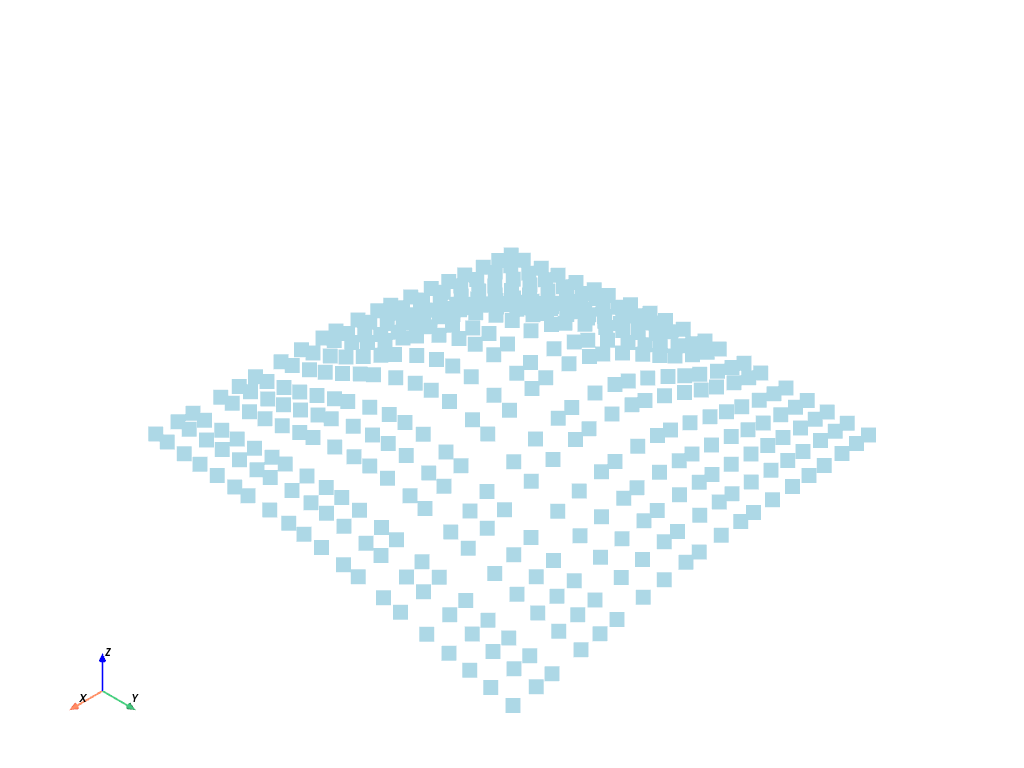Now that we have a PyVista data structure of the points, we can perform a triangulation to turn those boring discrete points into a connected surface. See https://docs.pyvista.org/api/core/_autosummary/pyvista.PolyData.delaunay_2d.html

```help(cloud.delaunay_2d)
```
```Help on method delaunay_2d in module pyvista.core.filters.poly_data:

delaunay_2d(tol=1e-05, alpha=0.0, offset=1.0, bound=False, inplace=False, edge_source=None, progress_bar=False) method of pyvista.core.pointset.PolyData instance
Apply a 2D Delaunay filter along the best fitting plane.

This filter can be used to generate a 2d surface from a set of
points on a plane.  If you want to create a surface from a
point cloud, see :func:`pyvista.PolyDataFilters.reconstruct_surface`.

Parameters
----------
tol : float, default: 1e-05
Specify a tolerance to control discarding of closely
spaced points. This tolerance is specified as a fraction
of the diagonal length of the bounding box of the points.

alpha : float, default: 0.0
Specify alpha (or distance) value to control output of
this filter. For a non-zero alpha value, only edges or
triangles contained within a sphere centered at mesh
vertices will be output. Otherwise, only triangles will be
output.

offset : float, default: 1.0
Specify a multiplier to control the size of the initial,
bounding Delaunay triangulation.

bound : bool, default: False
Boolean controls whether bounding triangulation points
and associated triangles are included in the
output. These are introduced as an initial triangulation
to begin the triangulation process. This feature is nice
for debugging output.

inplace : bool, default: False
If ``True``, overwrite this mesh with the triangulated
mesh.

edge_source : pyvista.PolyData, optional
Specify the source object used to specify constrained
edges and loops. If set, and lines/polygons are defined, a
constrained triangulation is created. The lines/polygons
are assumed to reference points in the input point set
(i.e. point ids are identical in the input and
source).

progress_bar : bool, default: False
Display a progress bar to indicate progress.

Returns
-------
pyvista.PolyData
Mesh from the 2D delaunay filter.

Examples
--------
First, generate 30 points on circle and plot them.

>>> import pyvista as pv
>>> points = pv.Polygon(n_sides=30).points
>>> circle = pv.PolyData(points)
>>> circle.plot(show_edges=True, point_size=15)

Use :func:`delaunay_2d` to fill the interior of the circle.

>>> filled_circle = circle.delaunay_2d()
>>> filled_circle.plot(show_edges=True, line_width=5)

Use the ``edge_source`` parameter to create a constrained delaunay
triangulation and plot it.

>>> squar = pv.Polygon(n_sides=4, radius=8, fill=False)
>>> squar = squar.rotate_z(45, inplace=False)
>>> circ0 = pv.Polygon(center=(2, 3, 0), n_sides=30, radius=1)
>>> circ1 = pv.Polygon(center=(-2, -3, 0), n_sides=30, radius=1)
>>> comb = circ0 + circ1 + squar
>>> tess = comb.delaunay_2d(edge_source=comb)
>>> tess.plot(cpos='xy', show_edges=True)

See :ref:`triangulated_surface` for more examples using this filter.
```

Apply the delaunay_2d filter

```surf = cloud.delaunay_2d()

# And plot it with edges shown
surf.plot(show_edges=True)
```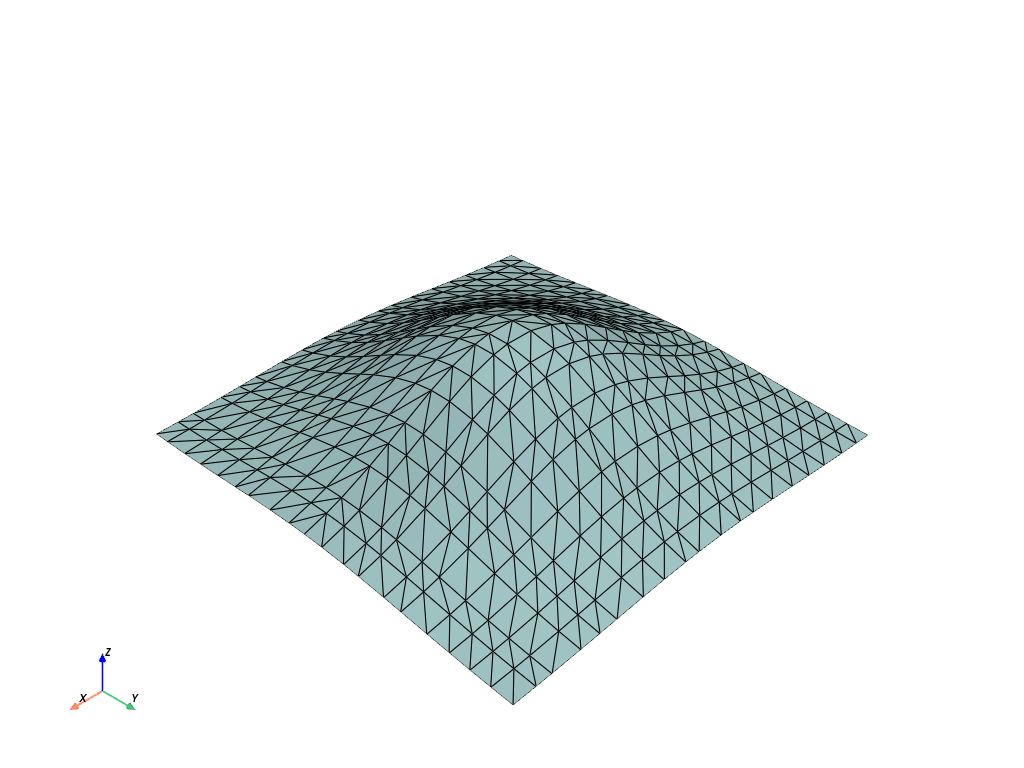## Clean Edges & Triangulations#

```# Create the points to triangulate
x = np.arange(10, dtype=float)
xx, yy, zz = np.meshgrid(x, x, )
points = np.column_stack((xx.ravel(order="F"), yy.ravel(order="F"), zz.ravel(order="F")))
# Perturb the points
points[:, 0] += np.random.rand(len(points)) * 0.3
points[:, 1] += np.random.rand(len(points)) * 0.3

# Create the point cloud mesh to triangulate from the coordinates
cloud = pv.PolyData(points)
cloud
```
PolyDataInformation
N Cells100
N Points100
N Strips0
X Bounds4.997e-02, 9.274e+00
Y Bounds5.451e-03, 9.276e+00
Z Bounds0.000e+00, 0.000e+00
N Arrays0

```cloud.plot(cpos="xy")
```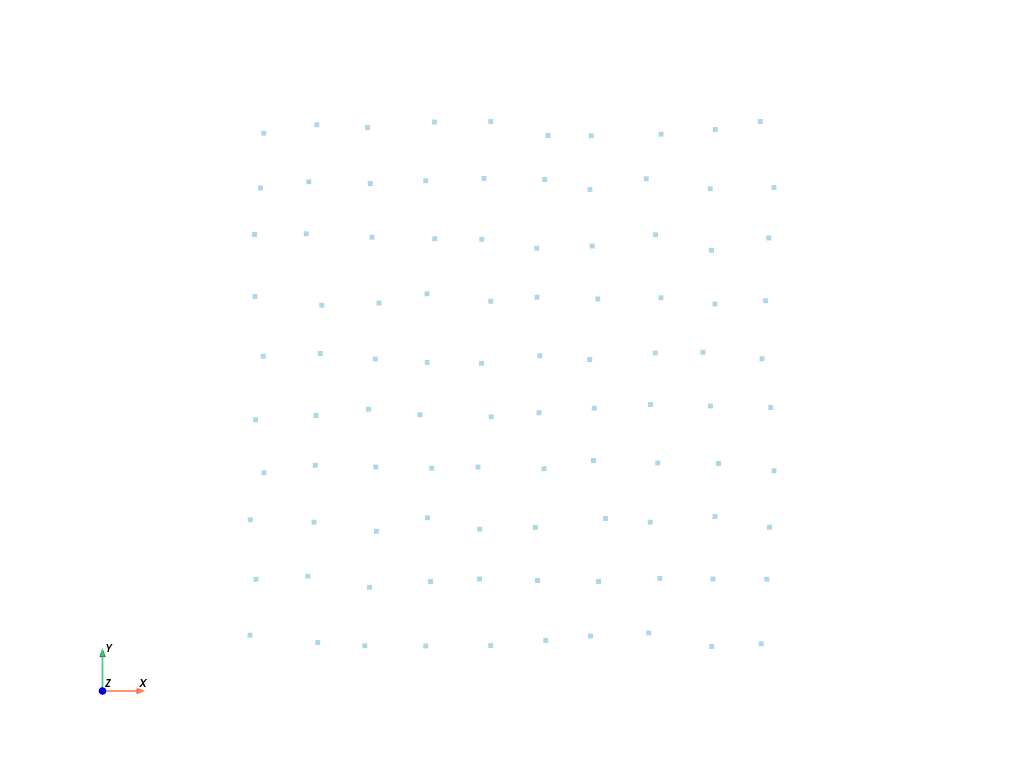Run the triangulation on these points

```surf = cloud.delaunay_2d()
surf.plot(cpos="xy", show_edges=True)
```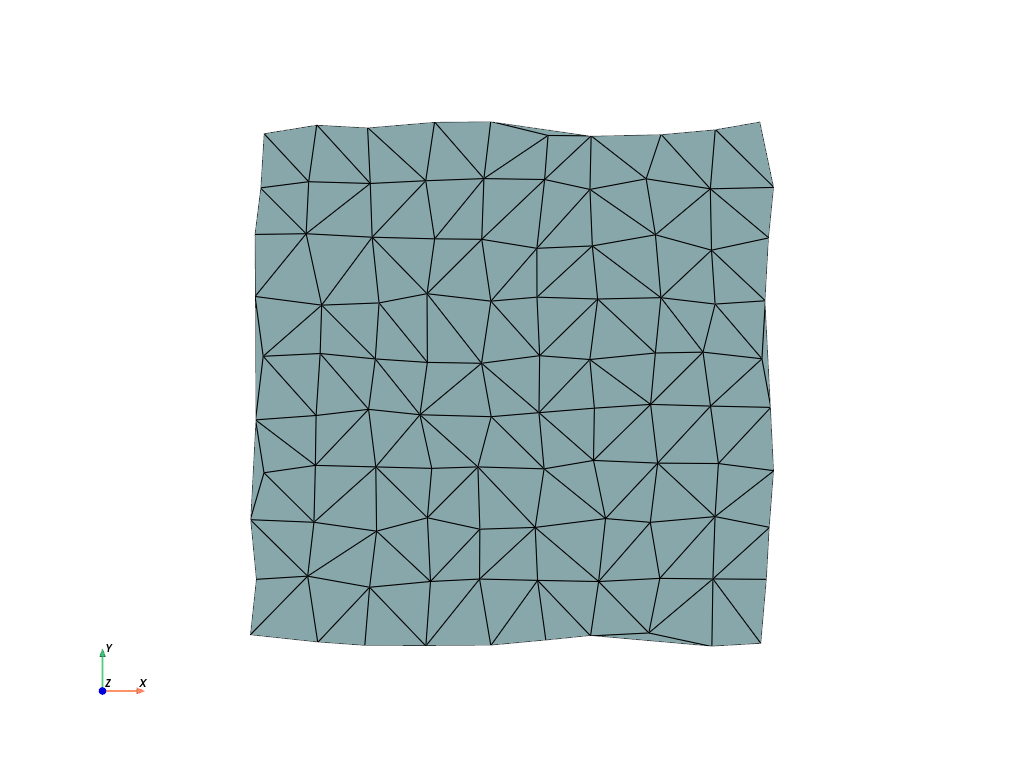Note that some of the outer edges are unconstrained and the triangulation added unwanted triangles. We can mitigate that with the `alpha` parameter.

```surf = cloud.delaunay_2d(alpha=1.0)
surf.plot(cpos="xy", show_edges=True)
```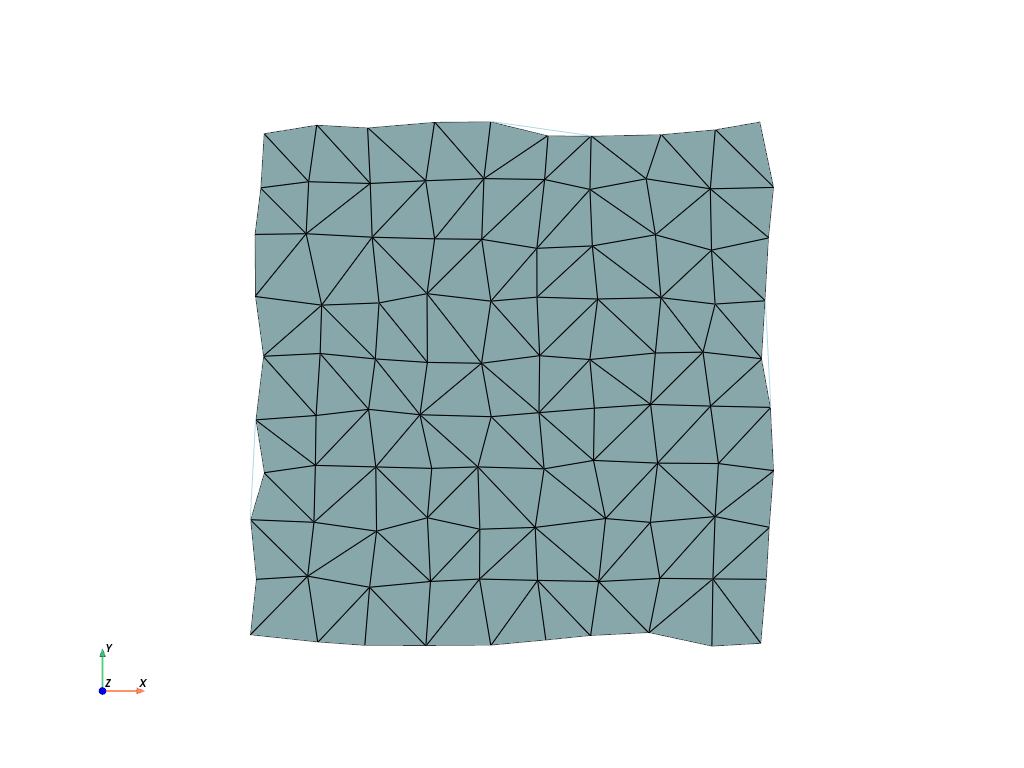Total running time of the script: ( 0 minutes 0.733 seconds)

Gallery generated by Sphinx-Gallery